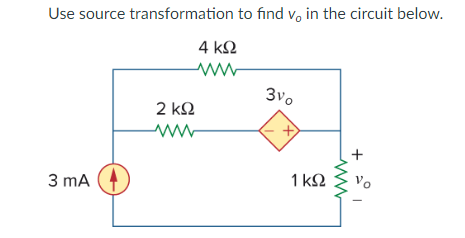Use source transformation to find V. in the circuit beloW3 mA1 ko

Question

Use source transformation to find vo in the circuit below. (Circuit is displayed in attached picture)help_outlineImage TranscriptioncloseUse source transformation to find V. in the circuit beloW 3 mA 1 ko fullscreen
Step 1

Consider the circuit diagram given, we can transform the voltage source with series resistor into an equivalent current source.

Then, the value of equivalent current source,

Step 2

The resulting circuit is shown below.

Step 3

Now, we can combine the two parallel resistors in the circuit.

The equi...

Want to see the full answer?

See Solution

Want to see this answer and more?

Our solutions are written by experts, many with advanced degrees, and available 24/7

See Solution
Tagged in

Electrical Engineering